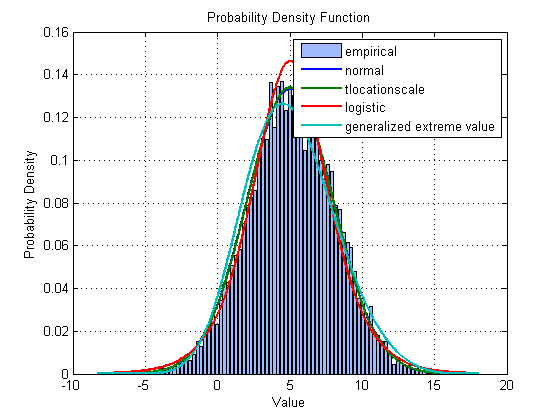# Finding the best distribution that fits the data1

Posted by Jiro Doke,

Jiro's pick this week is allfitdist by Mike Sheppard.

As an application engineer, I go out and deliver seminars on various topics (check out some upcoming events!), and one of the topics that seem to drum up a lot of interest is data modeling/fitting. It's a very broad topic and spans pretty much all industries. People are always trying to model some phenomena so that they can use them to make predictions, understand characteristics, or optimize. The techniques people can use vary based on what they are trying to model.

Probably the most common technique is parametric modeling, where you know the form (equation) of the model. There are different ways of doing this in MATLAB, including commands like polyfit and the back slash operator. There are many other ways that span various techniques covered by toolboxes, such as Curve Fitting Toolbox, Statistics Toolbox, and Optimization Toolbox.

During one of my seminars on these modeling techniques, a user came up to me and asked me if it was possible to get an equation just by providing some data (inputs and outputs). This is not possible without any assumptions on the model, but I hear this question from time to time. When I dig in a little bit, it turns out that, most of the time, people have some idea for the form of the model, like power series, etc. But if they truly want a black-box model, there are plenty of techniques out there for doing that, such as decision tree learning (1), artificial neural networks (2), and system identification (3).

Back to the story... The question from this user at the seminar generated a healthy discussion back at the office on how to address this type of question. The key is that there is virtually an infinite number of equations that could describe a data set. Without any constraints on the form, it's impossible to return a single equation. But then one of my colleagues pointed out that this type of question may be more reasonable when it is about distribution fitting. It's a much smaller scope and there may be a finite set of distributions that could be tested.

This is where Mike's allfitdist comes into play. Statistics Toolbox supports a long list of distributions, including parametric and nonparametric distributions. allfitdist fits all valid parametric distributions to the data and sorts them using a metric you can use to compare the goodness of the fit.

Here's an example of finding the best distribution fit for a random data set with an assumed unknown continuous distribution (mu=5, sigma=3).

% Create a normally distributed (mu: 5, sigma: 3) random data set
x = normrnd(5, 3, 1e4, 1);

% Compute and plot results. The results are sorted by "Bayesian information
% criterion".
[D, PD] = allfitdist(x, 'PDF');And the best fit is...

D(1)
ans =
DistName: 'normal'
NLogL: 2.5148e+004
BIC: 5.0314e+004
AIC: 5.0300e+004
AICc: 5.0300e+004
ParamNames: {'mu'  'sigma'}
ParamDescription: {'location'  'scale'}
Params: [5.0093 2.9918]
Paramci: [2x2 double]
ParamCov: [2x2 double]
Support: [1x1 struct]


Notice that it found the normal distribution as the best fit and the parameters (mu and sigma) to be close to the actual.

fprintf('%10s\t'  , D(1).ParamNames{:}); fprintf('\n');
fprintf('%10.2f\t', D(1).Params       ); fprintf('\n');
        mu	     sigma
5.01	      2.99


The function is very well documented with good examples. This is definitely a must-have for anyone doing statistical analysis of real-world data. Give it a try and let us know what you think here or leave a comment for Mike.

Get the MATLAB code

Published with MATLAB® 7.13